Project

# EFM8 Sound Synthesizer: From Square Wave to Sine Wave

September 04, 2015 by Robert Keim

## Part 1 in the “How to Make an EFM8-Based Sound Synthesizer” series, which will design a sound synthesizer that can play standard musical notes. We will accomplish this using a low-cost, small-form-factor EFM8 microcontroller in conjunction with a few external components and a speaker.

Part 1 in the “How to Make an EFM8-Based Sound Synthesizer” series.

Intermediate

#### Required Hardware/Software

 Description Quantity Digi-Key p/n Breadboard 1 377-2094-ND Receptacle-to-plug jumper wires 4 1471-1231-ND 5 V AC/DC wall-mount power supply 1 1470-2771-ND 0.1 µF capacitors 2 399-4266-ND Fifth-order switched-capacitor lowpass filter 1 LTC1063CN8#PBF-ND

#### Project Overview

The goal of this project is to design a sound synthesizer that can play standard musical notes. We will accomplish this using a low-cost, small-form-factor EFM8 microcontroller in conjunction with a few external components and a speaker. In this first article we will focus on converting a square wave generated by the EFM8 into a sine wave suitable for driving a speaker. The essential component in this process is a clock-tunable monolithic lowpass filter. In this project we will use the LTC1063 fifth-order Butterworth filter IC from Linear Technology, though parts with higher-order frequency response and different filter types are available (such as the MAX7401, an eighth-order Bessel lowpass filter from Maxim Integrated). ICs such as these are based on switched-capacitor filter designs, so we will start with a brief overview of this technology.

#### Replacing Resistors

The essence of a switched-capacitor filter topology is replacing resistors with switches that open and close according to a specific pattern.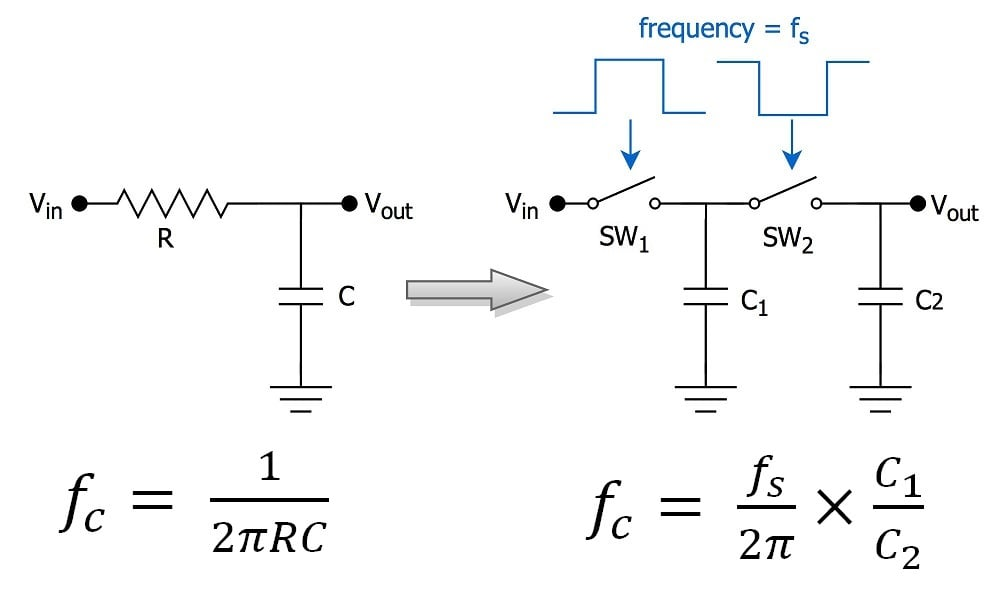On the left-hand side of the above diagram is a standard RC lowpass filter, and on the right is the switched-capacitor equivalent. As you can see, the resistor has been replaced by a combination of two switches and a capacitor (the latter being the eponymous “switched capacitor”). The concept is as follows: When SW1 is closed and SW2 is open, the input voltage charges C1. Next, when SW1 is open and SW2 is closed, charge moves from C1 through SW2 to the output. What we have here is electric charge moving from input to output. The amount of charge transferred per clock cycle is proportional to the capacitance of C1 and the difference between Vin and Vout, and the amount of charge transferred per second is thus proportional to C1, Vin-Vout, and the number of clock cycles per second, i.e., frequency. If we recall that current is the amount of charge per unit time flowing through a conductor, we can see that the switched-capacitor arrangement produces an average current given as follows: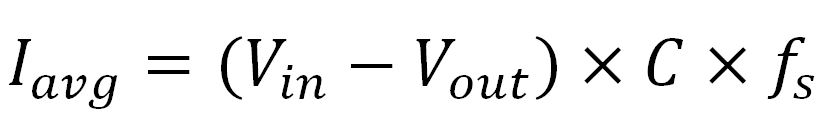We know from Ohm’s law that the voltage across a component divided by the current flowing through that component equals the resistance of the component, and thus the equivalent resistance of the switched-capacitor circuit is the following: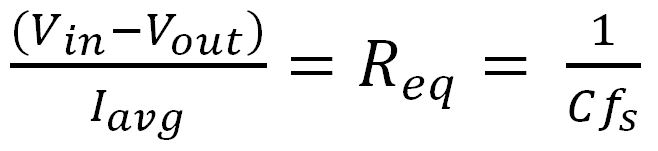This is significant: the value of this equivalent resistance can be adjusted simply by varying the frequency of the clock that drives the switches, and thus the cutoff frequency of a switched-capacitor RC filter can be controlled by the frequency of a digital square wave. Furthermore, the cutoff frequency depends not on an absolute capacitance value but rather on the ratio of C1 to C2, and IC manufacturing processes can more effectively control the ratio between component values than the component values themselves. Thus, the switched-capacitor filter topology is versatile and highly compatible with IC technology. IC manufacturers can incorporate multiple switched-capacitor-based filter stages into a single chip, resulting in high-order filters with a frequency response that can be conveniently and precisely controlled by the frequency of a digital square wave.

#### From Square to Sine

The Fourier transform of a square wave with amplitude k and frequency f is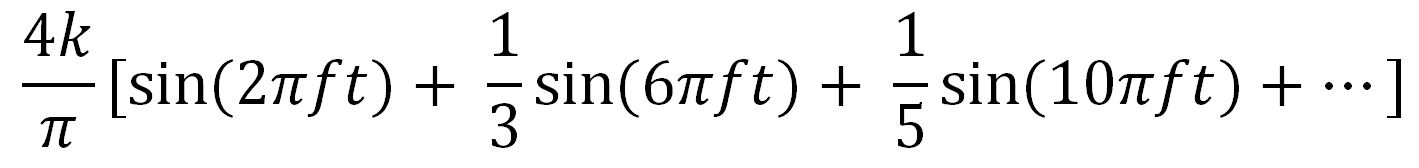In other words, a square wave is the sum of an infinite series of sine waves with frequency increasing and amplitude decreasing by a factor of 3, 5, 7, 9, and so forth. These frequency components are referred to as odd-integer harmonics. (You can find a detailed discussion of this topic here.) The first term in this series has the same peak amplitude and frequency as the original square wave, so this is the term we want. If we can filter out all the others, we will have a smooth, audio-friendly sine wave instead of a clunky square wave.

This is where the fifth-order lowpass filter comes in. We should get 6 dB/octave (or 20 dB/decade) of roll-off for each pole in the frequency response, so the theoretical roll-off of our five-pole system is 30 dB per octave. The frequency response curve given in the LTC1063 datasheet confirms this: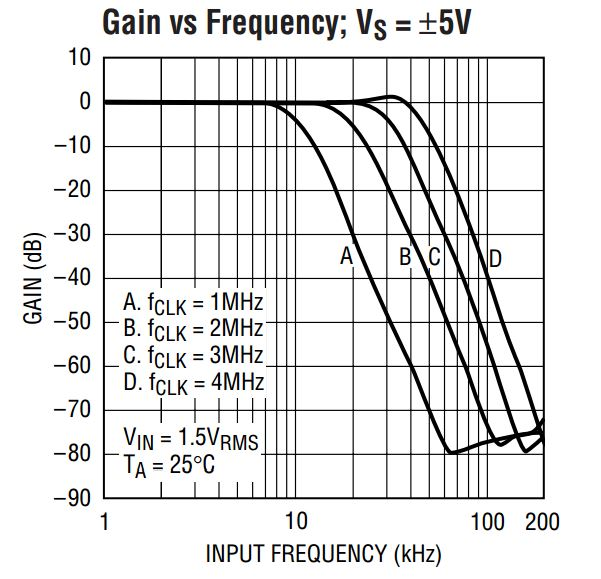An octave corresponds to a doubling of frequency, and we can see that from 20 kHz to 40 kHz the gain decreases by 30 dB. The frequency of the second term in the Fourier series given above is three times the square wave frequency; thus, with proper placement of the filter’s cutoff frequency, this harmonic should be attenuated by 40 or maybe 45 dB. Attenuation of 40 dB corresponds to a factor-of-100 reduction in amplitude, and higher harmonics will be attenuated even more (by at least 70 dB), so at this point it looks like the LTC1063 will do a fine job of turning our square wave into a sine wave.

#### Firmware Overview

The EFM8 needs to generate two square waves of different frequency. The first is the same frequency as the sine wave that will drive the speaker, and the second drives the LTC1063’s clock pin and thereby controls the filter’s cutoff frequency. The LTC1063’s clock-to-cutoff ratio is 100 to 1; in other words, the cutoff frequency equals the clock frequency divided by 100. Be careful, though—this does not mean that the frequency ratio between the two signals generated by the EFM8 should be 100 to 1. Why? Consider the following plot, which provides detailed information about the filter’s frequency response near the cutoff frequency: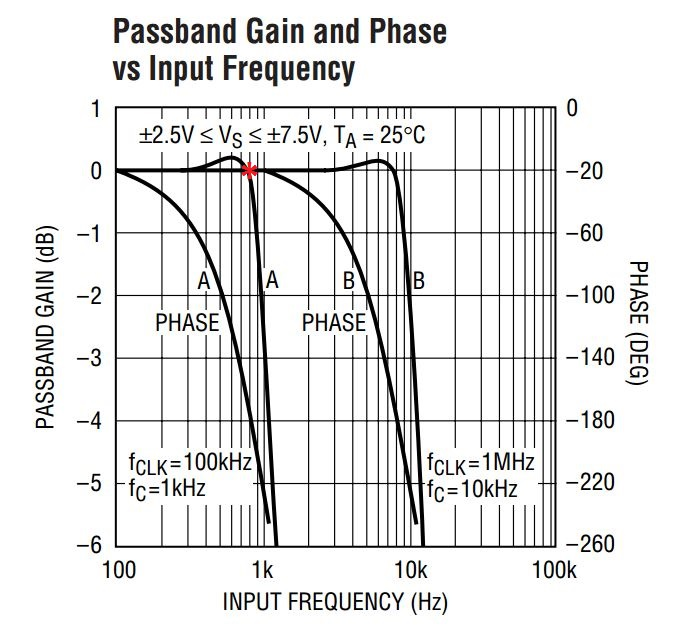Recall that another name for the cutoff frequency is the 3-dB frequency: at the cutoff frequency, the gain is -3 dB, not 0 dB. So if the EFM8’s clock-signal frequency is exactly 100 times the sound-signal frequency, the sound signal will be attenuated by 3 dB. Instead, we want the sound signal frequency to be located right where the frequency response begins to roll off; this location is identified by the red asterisk in the above plot. The frequency of this point is about 800 Hz; the clock frequency for this plot is 100 kHz, and 100 kHz divided by 800 Hz equals 125. Thus, we will configure the EFM8 for a clock-signal-to-sound-signal ratio of 125 to 1.

#### Peripherals, Ports, and Interrupts

We will generate the two square waves using the EFM8’s programmable counter array (PCA). This peripheral consists of three channels that are driven by the same clock but otherwise function independently. Each PCA channel can perform a variety of timing and waveform-generation tasks; in this case we will configure PCA channel 0 and 1 for “high speed output” mode: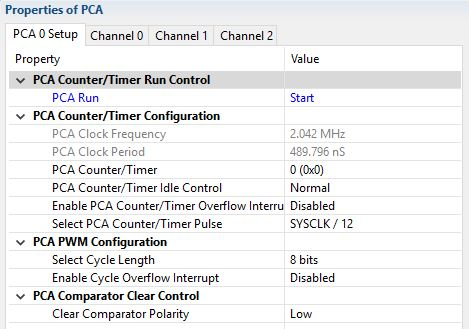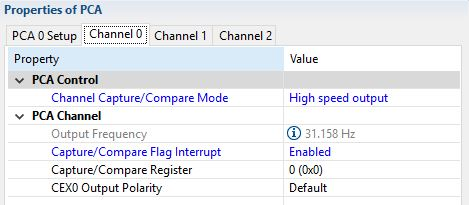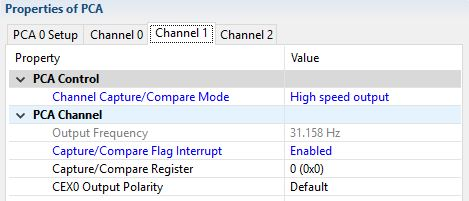Note that the “output frequency” shown above is not relevant because we will control the frequency by manually updating each channel’s capture/compare register (download code at bottom of page):


/**************************************
output frequency timing
**************************************/
/*PCA clock period was measured at 488 ns.
For a 400 Hz output, the period is 2.5 ms.
2.5 ms divided by 488 ns = 5123.
So, 5123 PCA clocks gives the proper period for a 400 Hz output.
However, we need to add only half of this value to the capture/compare
register because a match between the capture/compare
register and the PCA counter causes the output pin to toggle,
and one clock period requires two toggles.
The frequency of the clock that drives the lowpass filter is 125 times
higher than the frequency of the sound signal, which means that the
period is 125 times shorter. Thus, we divide the sound-signal increment
by 125 to obtain the filter-clock increment.
This same procedure is used to calculate the sound-signal and
clock-signal increments for a 600 Hz output.*/
#define SOUND_400Hz_INCREMENT 2562
#define FILTCLK_400Hz_INCREMENT 20
#define SOUND_600Hz_INCREMENT 1708
#define FILTCLK_600Hz_INCREMENT 14



The timing details are given in the comments included in the code excerpt. The general concept is as follows: The 16-bit PCA counter is always incrementing; when it reaches 0xFFFF, it overflows and keeps on counting. This counter is used for all three PCA channels. Each channel has its own capture/compare register. In “high speed output” mode, the PCA channel toggles its output pin every time the 16-bit value of the PCA counter is equal to the 16-bit value stored in the channel’s capture/compare register. By repeatedly incrementing the capture/compare register with a predetermined value, we can precisely control the amount of time between successive capture/compare matches, and this is where the PCA interrupt comes in: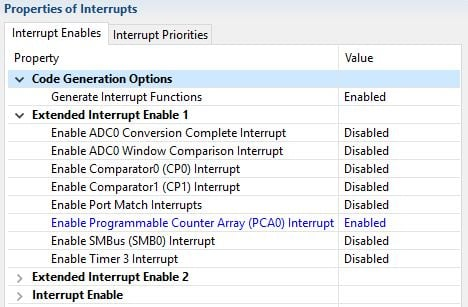Now, every time there is a match between the PCA counter and the channel’s capture/compare register, the output pin will toggle and an interrupt will fire. In the interrupt service routine, we control the period of the output signal by adding the predetermined increment to the previous capture/compare value:


SI_INTERRUPT (PCA0_ISR, PCA0_IRQn)
{
if(PCA0CN0_CCF0)	//this channel generates the sound-signal frequency
{
PCA0CN0_CCF0 = 0;		//clear the interrupt flag
PCA0Mod0_Compare_Value = PCA0Mod0_Compare_Value + Current_SoundSignal_Increment;
PCA0CPL0 = LOWBYTE(PCA0Mod0_Compare_Value);	//must write low byte first
PCA0CPH0 = HIGHBYTE(PCA0Mod0_Compare_Value);
}

if(PCA0CN0_CCF1)	//this channel generates the filter-clock frequency
{
PCA0CN0_CCF1 = 0;		//clear the interrupt flag
PCA0Mod1_Compare_Value = PCA0Mod1_Compare_Value + Current_FilterClock_Increment;
PCA0CPL1 = LOWBYTE(PCA0Mod1_Compare_Value);	//must write low byte first
PCA0CPH1 = HIGHBYTE(PCA0Mod1_Compare_Value);
}
}



Note that we are using unsigned 16-bit variables here, so we don’t have to worry about manually dealing with variable overflow: when the increment causes the PCA0Mod0/1_Compare_Value variables to exceed 65535, they will automatically overflow to the correct value because the PCA counter is also 16 bits.

The two PCA channel output signals are driven on P0.0 and P1.1: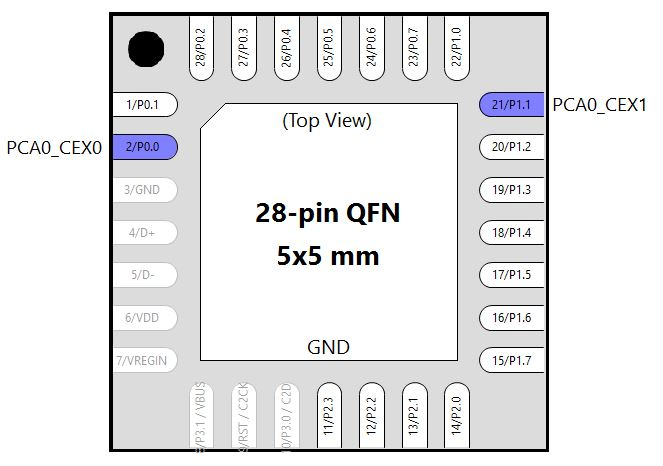The only other peripheral needed for the current firmware is Timer3, which is used for delays on the order of milliseconds to seconds: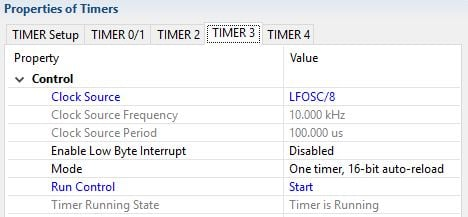We also need to enable the low-frequency oscillator so that we can use it as the clock source for Timer3: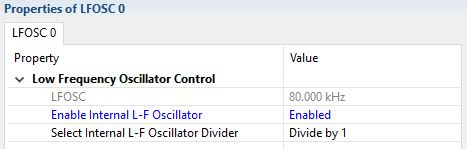#### Circuit

Here is the schematic for this portion of the project: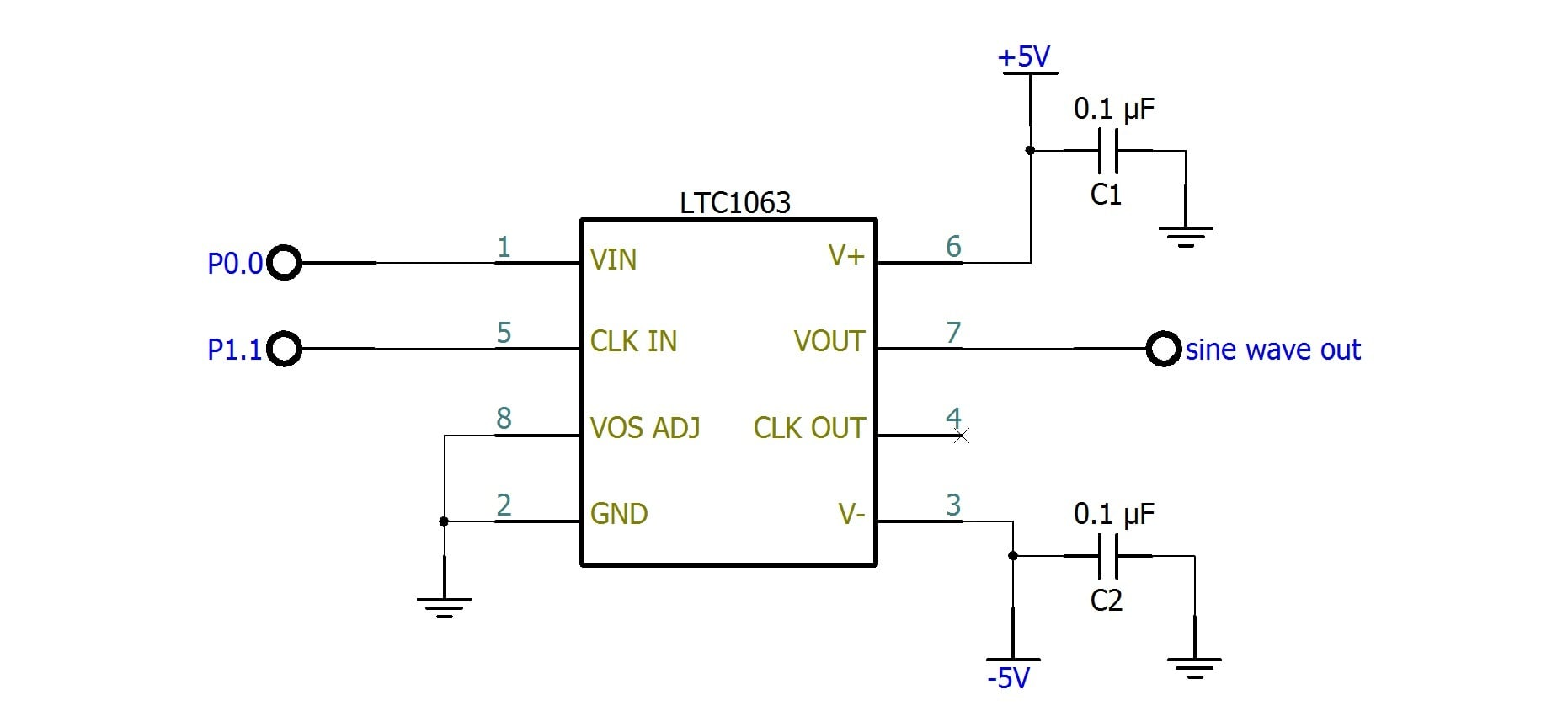And here is the breadboard implementation: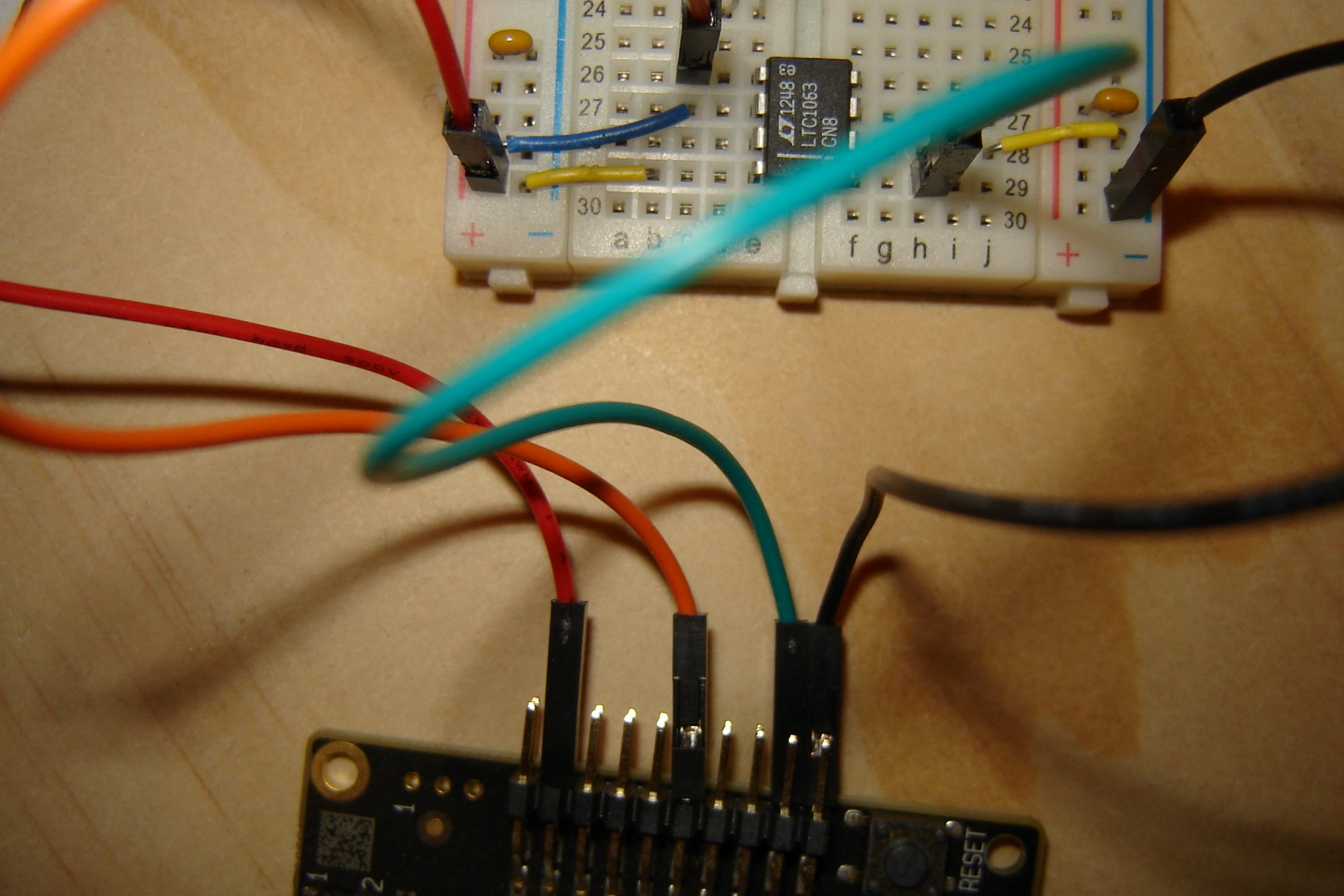Here is the main while loop for this firmware:


while(1)
{
Current_SoundSignal_Increment = SOUND_400Hz_INCREMENT;
Current_FilterClock_Increment = FILTCLK_400Hz_INCREMENT;

//delay 3 seconds
SFRPAGE = TIMER3_PAGE; TMR3 = 0; while(TMR3<30000);

Current_SoundSignal_Increment = SOUND_600Hz_INCREMENT;
Current_FilterClock_Increment = FILTCLK_600Hz_INCREMENT;

//delay 3 seconds
SFRPAGE = TIMER3_PAGE; TMR3 = 0; while(TMR3<30000);
}



SoundSynthesizer_Part1.zip

In this infinite loop, the EFM8 outputs the sound and filter-clock signals for a 400 Hz sine wave and holds for three seconds, then it switches to sound and filter-clock signals for a 600 Hz sine wave and again holds for three seconds. The video at the end of this article shows the square wave generated by the EFM8 and the sine wave available at the output of the LTC1063. The oscilloscope’s frequency measurement functionality (in the bottom-right portion of the screen under “CH1 Freq”) confirms that the generated signals are indeed 400 and 600 Hz.

Some additional filtering and buffering circuitry is needed before we use these sine wave signals to drive a speaker, and this is what we will explore in the next article.

Next Article in Series: EFM8 Sound Synthesizer: Driving the Speaker

Give this project a try for yourself! Get the BOM.